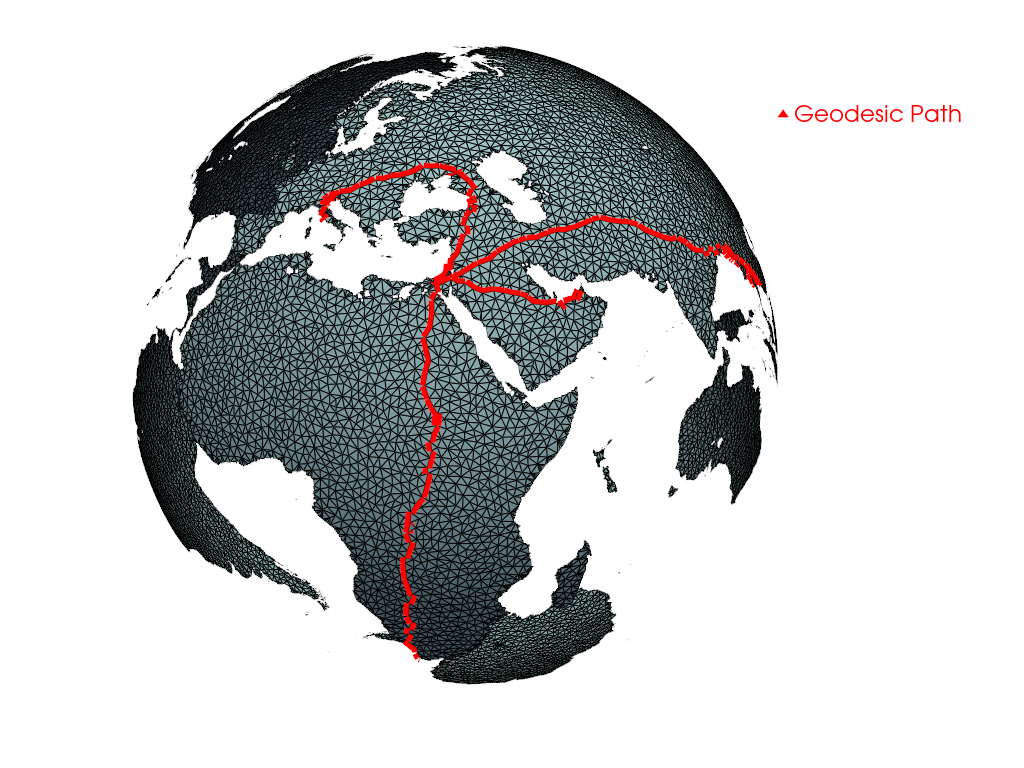# Geodesic Paths¶

Calculates the geodesic path between two vertices using Dijkstra’s algorithm

```# sphinx_gallery_thumbnail_number = 1
import pyvista as pv
from pyvista import examples

```

Get the geodesic path as a new `pyvista.PolyData` object:

```geodesic = sphere.geodesic(0, sphere.n_points - 1)
```

Render the path along the sphere

```p = pv.Plotter()
p.camera_position = [-1, -1, 1]
p.show()
```Out:

```[(-24603707957.038784, -24590884070.56062, 24590884070.56062),
(-12823886.478166103, 4.76837158203125e-07, 0.0),
(0.0, 0.0, 1.0)]
```

How long is that path?

```distance = sphere.geodesic_distance(0, sphere.n_points - 1)
distance
```

Out:

```19973118631.340134
```

Total running time of the script: ( 0 minutes 1.551 seconds)

Gallery generated by Sphinx-Gallery